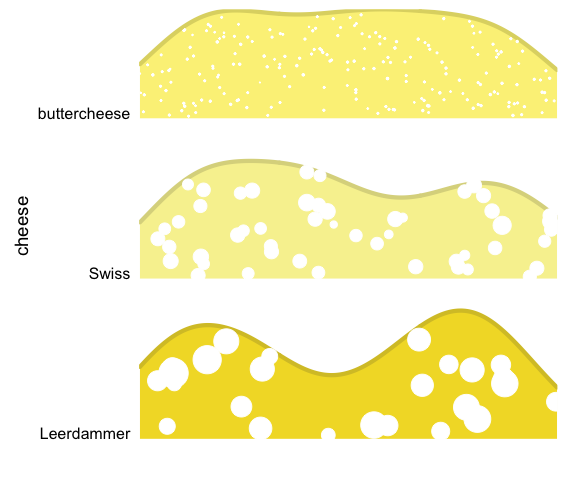# Gallery of ggridges examples

## Evolution of movie lengths over time

Data from the IMDB, as provided in the ggplot2movies package.

library(ggplot2movies)
ggplot(movies[movies$year>1912,], aes(x = length, y = year, group = year)) + geom_density_ridges(scale = 10, size = 0.25, rel_min_height = 0.03) + theme_ridges() + scale_x_continuous(limits=c(1, 200), expand = c(0.01, 0)) + scale_y_reverse(breaks=c(2000, 1980, 1960, 1940, 1920, 1900), expand = c(0.01, 0))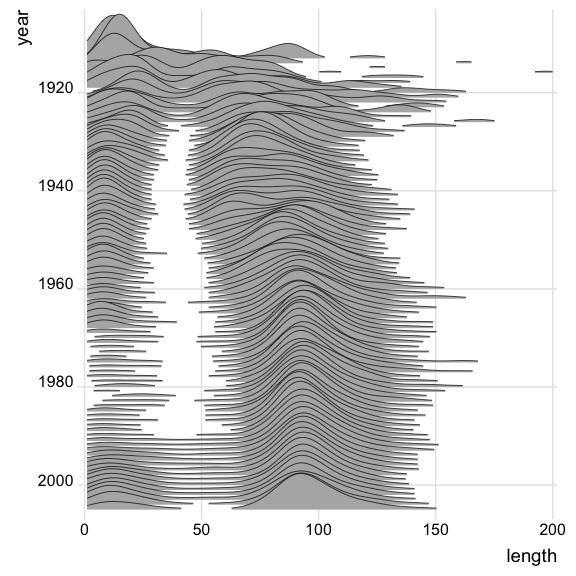## Results from Catalan regional elections, 1980-2015 Modified after a figure originally created by Marc Belzunces. library(dplyr) library(forcats) Catalan_elections %>% mutate(YearFct = fct_rev(as.factor(Year))) %>% ggplot(aes(y = YearFct)) + geom_density_ridges( aes(x = Percent, fill = paste(YearFct, Option)), alpha = .8, color = "white", from = 0, to = 100 ) + labs( x = "Vote (%)", y = "Election Year", title = "Indy vs Unionist vote in Catalan elections", subtitle = "Analysis unit: municipalities (n = 949)", caption = "Marc Belzunces (@marcbeldata) | Source: Idescat" ) + scale_y_discrete(expand = c(0.01, 0)) + scale_x_continuous(expand = c(0.01, 0)) + scale_fill_cyclical( breaks = c("1980 Indy", "1980 Unionist"), labels = c(1980 Indy = "Indy", 1980 Unionist = "Unionist"), values = c("#ff0000", "#0000ff", "#ff8080", "#8080ff"), name = "Option", guide = "legend" ) + theme_ridges(grid = FALSE)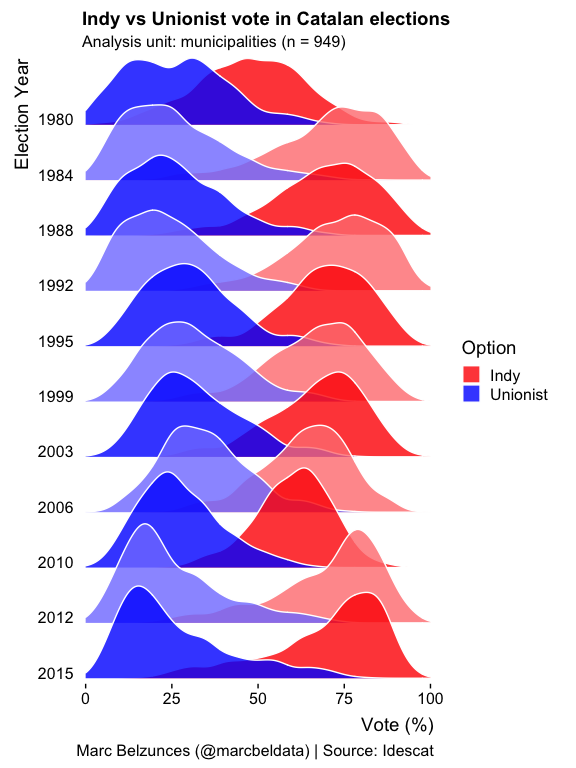## Temperatures in Lincoln, Nebraska Modified from a blog post by Austin Wehrwein. library(viridis) ggplot(lincoln_weather, aes(x = Mean Temperature [F], y = Month, fill = ..x..)) + geom_density_ridges_gradient(scale = 3, rel_min_height = 0.01, gradient_lwd = 1.) + scale_x_continuous(expand = c(0.01, 0)) + scale_y_discrete(expand = c(0.01, 0)) + scale_fill_viridis(name = "Temp. [F]", option = "C") + labs( title = 'Temperatures in Lincoln NE', subtitle = 'Mean temperatures (Fahrenheit) by month for 2016\nData: Original CSV from the Weather Underground' ) + theme_ridges(font_size = 13, grid = TRUE) + theme(axis.title.y = element_blank())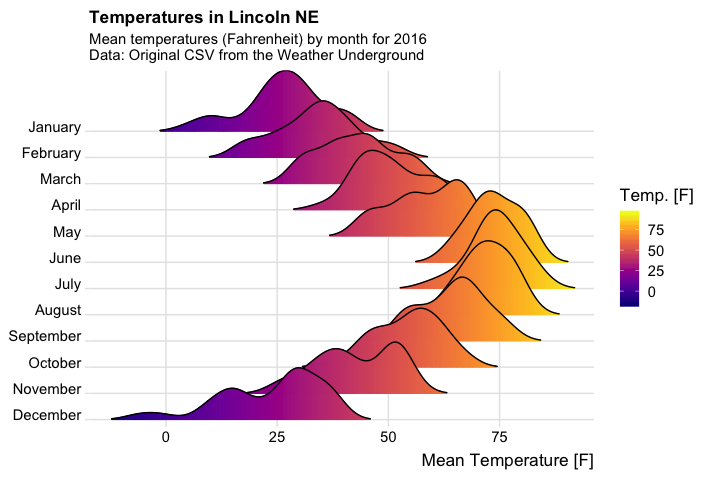## Visualization of Poisson random samples with different means Inspired by a ggridges example by Noam Ross. # generate data set.seed(1234) pois_data <- data.frame(mean = rep(1:5, each = 10)) pois_data$group <- factor(pois_data$mean, levels=5:1) pois_data$value <- rpois(nrow(pois_data), pois_data$mean) # make plot ggplot(pois_data, aes(x = value, y = group, group = group)) + geom_density_ridges2(aes(fill = group), stat = "binline", binwidth = 1, scale = 0.95) + geom_text( stat = "bin", aes( y = group + 0.95*(..count../max(..count..)), label = ifelse(..count..>0, ..count.., "") ), vjust = 1.4, size = 3, color = "white", binwidth = 1 ) + scale_x_continuous( breaks = c(0:12), limits = c(-.5, 13), expand = c(0, 0), name = "random value" ) + scale_y_discrete( expand = c(0.01, 0), name = "Poisson mean", labels = c("5.0", "4.0", "3.0", "2.0", "1.0") ) + scale_fill_cyclical(values = c("#0000B0", "#7070D0")) + labs( title = "Poisson random samples with different means", subtitle = "sample size n=10" ) + guides(y = "none") + theme_ridges(grid = FALSE) + theme( axis.title.x = element_text(hjust = 0.5), axis.title.y = element_text(hjust = 0.5) )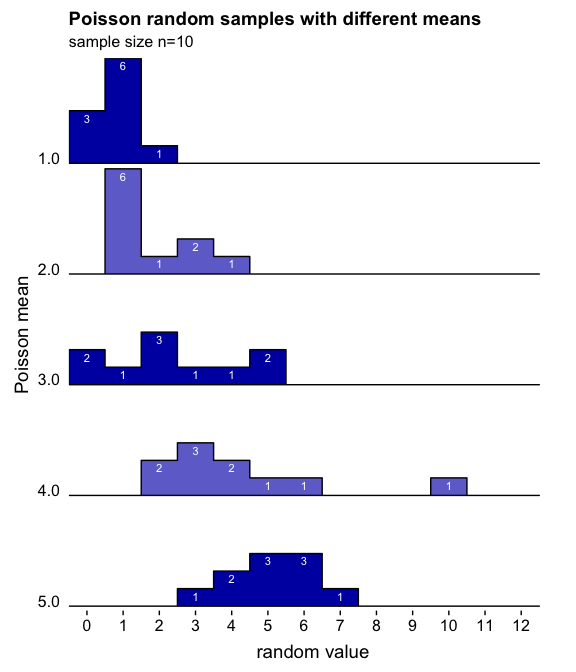## Height of Australian athletes library(DAAG) # for ais dataset ais$sport <- factor(
ais\$sport,
levels = c("B_Ball", "Field", "Gym", "Netball", "Row", "Swim", "T_400m", "T_Sprnt", "Tennis", "W_Polo"),
labels = c("Basketball", "Field", "Gym", "Netball", "Row", "Swim", "Track 400m", "Track Sprint", "Tennis", "Water Polo")
)

ggplot(ais, aes(x=ht, y=sport, color=sex, point_color=sex, fill=sex)) +
geom_density_ridges(
jittered_points=TRUE, scale = .95, rel_min_height = .01,
point_shape = "|", point_size = 3, size = 0.25,
position = position_points_jitter(height = 0)
) +
scale_y_discrete(expand = c(.01, 0)) +
scale_x_continuous(expand = c(0, 0), name = "height [cm]") +
scale_fill_manual(values = c("#D55E0050", "#0072B250"), labels = c("female", "male")) +
scale_color_manual(values = c("#D55E00", "#0072B2"), guide = "none") +
scale_discrete_manual("point_color", values = c("#D55E00", "#0072B2"), guide = "none") +
guides(fill = guide_legend(
override.aes = list(
fill = c("#D55E00A0", "#0072B2A0"),
color = NA, point_color = NA))
) +
ggtitle("Height in Australian athletes") +
theme_ridges(center = TRUE)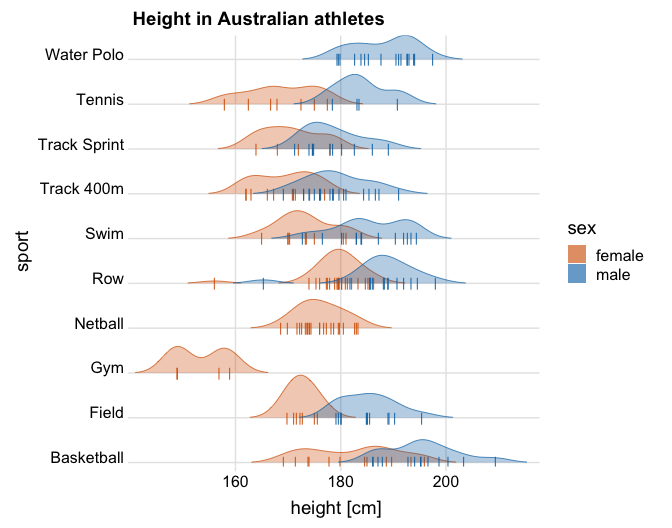## A cheese plot

Inspired by this tweet by Leonard Kiefer.

set.seed(423)
n1 <- 200
n2 <- 25
n3 <- 50
cols <- c('#F2DB2F', '#F7F19E', '#FBF186')
cols_dark <- c("#D7C32F", "#DBD68C", "#DFD672")
cheese <- data.frame(
cheese = c(rep("buttercheese", n1), rep("Leerdammer", n2), rep("Swiss", n3)),
x = c(runif(n1), runif(n2), runif(n3)),
size = c(rnorm(n1, mean = .1, sd = .01), rnorm(n2, mean = 9, sd = 3), rnorm(n3, mean = 3, sd = 1))
)
ggplot(cheese, aes(x = x, point_size = size, y = cheese, fill = cheese, color = cheese)) +
geom_density_ridges(
jittered_points = TRUE, point_color="white", scale = .8, rel_min_height = .2,
size = 1.5
) +
scale_y_discrete(expand = c(.01, 0)) +
scale_x_continuous(limits = c(0, 1), expand = c(0, 0), name = "", breaks = NULL) +
scale_point_size_continuous(range = c(0.01, 10), guide = "none") +
scale_fill_manual(values = cols, guide = "none") +
scale_color_manual(values = cols_dark, guide = "none") +
theme_ridges(grid = FALSE, center = TRUE)